# Chapter 9 of 24 - Multiplicative Functions

Multiplication! That sounds nice and simple doesn't it! Unfortunately, that's not what that title means.

Any number can be broken down into its prime decomposition (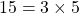). A multiplicative function is where a function of a number is equal to the function of each prime in the prime decomposition of that number all multiplied together. So if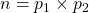, where p is a prime in the prime decomposition and if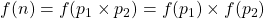, then the function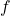is a multiplicative function.

Next section went into detail about perfect numbers. A perfect number is equal to the sum of its positive factors excluding itself. Best example here is the perfect number 6=1+2+3.

The rest of the chapter was introducing Euler's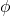-function (also multiplicative), and using this new function, the introduction of primitive roots.